# Excel XOR Function

## XOR Function

The XOR function is a premade function in Excel, which returns TRUE or FALSE based on two or more conditions.

Note: The XOR function is often used together with the IF function.

It is typed `=XOR`:

=XOR([logical1], [logical2], ...)

The conditions are referred to as `[logical1]`, `[logical2]`, and so on.

The conditions can check things like:

• If a number is greater than another number `>`
• If a number is smaller than another number `<`
• If a number or text is equal to something `=`

For two conditions, you can think of the XOR function as: "either this or that, but not both"

Note: The different parts of the function are separated by a symbol, like comma `,` or semicolon `;`

The symbol depends on your Language Settings.

## Example XOR Function

Check if the Pokemon type is either Fire type OR has HP less than 60, but not both:

The function returns "TRUE" or "FALSE".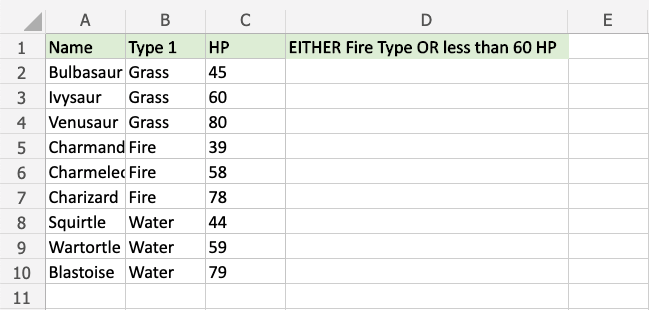Copy Values

Example XOR function, step by step:

1. Select the cell `D2`
2. Type `=XOR`
3. Double click the XOR command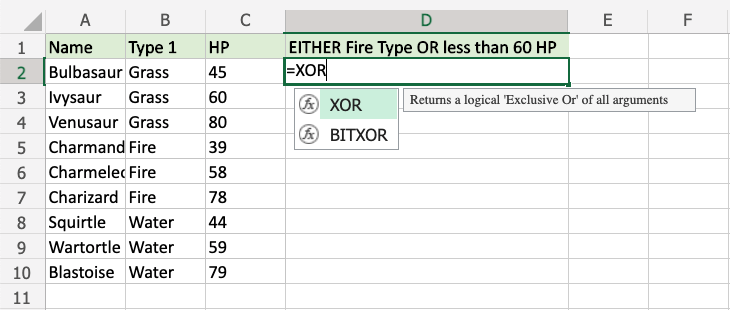1. Specify the first condition `B2="Fire"`
2. Type `,`
3. Specify the second condition `C2<60`
4. Hit enter

Note: You can add more conditions by repeating steps 5-6 before hitting enter.

The XOR function returns "TRUE" if an odd number (1,3,5,..) of conditions are TRUE.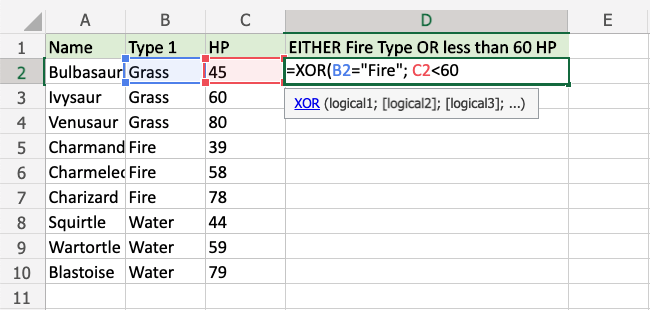Since the value in cell `B2` is not "Fire" the first condition is FALSE.

Since the value in cell `C2` is less than 60 the second condition is also FALSE.

For two conditions, only one condition should to be TRUE for the XOR function to return TRUE.

Note: Text values needs to be in quotes: " "

The function can be repeated with the filling function for each row to perform the same check for each Pokemon: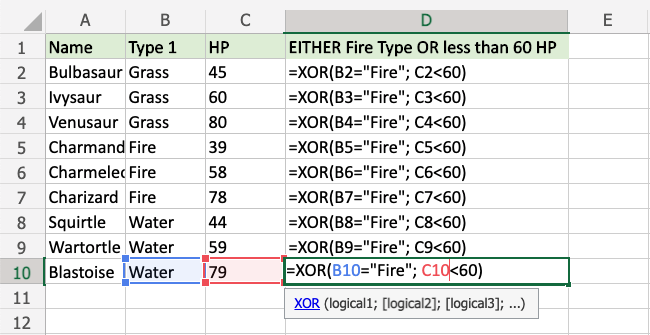Now, each row has a check for Fire Type or HP less than 60, but not both: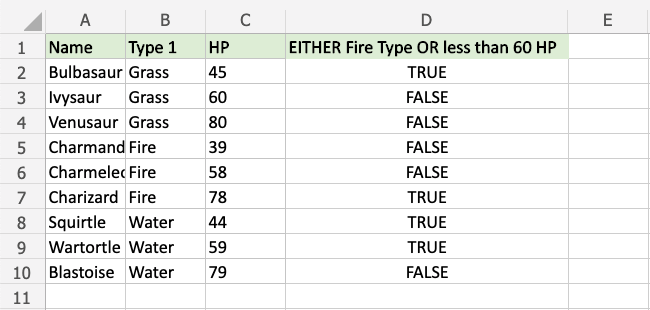For example:

• Ivysaur is not Fire type, but it also does not have less than 60 defense, so the function returns "FALSE".
• Charmeleon is Fire type, and has less than 60 defense, so the function returns "FALSE"
• Wartortle is not Fire type, but has less than 60 defense, so the function returns "TRUE"

## Example XOR Function (with IF)

Combining the XOR function with an IF function lets you check multiple conditions for the IF function:

Note: The IF function lets you specify the return values.

The IF function is typed `=IF` and has 3 parts:

=IF(logical_test, [value_if_true], [value_if_false])

The XOR function takes the place of the `logical_test` condition.

Here, the IF function returns "Yes" or "No".

Example XOR function as the condition for IF function, step by step:

1. Select cell `C2`
2. Type `=IF`
3. Double click the IF command1. Type `XOR`
2. Double click the XOR command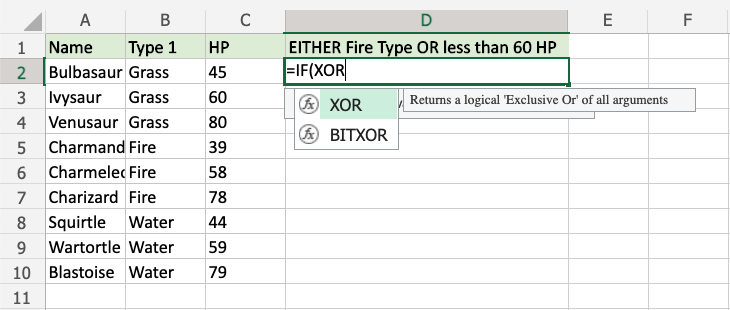1. Specify the first condition `B2="Fire"`
2. Type `,`
3. Specify the second condition `C2<60`
4. Type `),`
5. Specify the value `"Yes"` for when just one condition is TRUE
6. Type `,`
7. Specify the value `"No"` for when both are FALSE, or TRUE at the same time
8. Type `)` and hit enter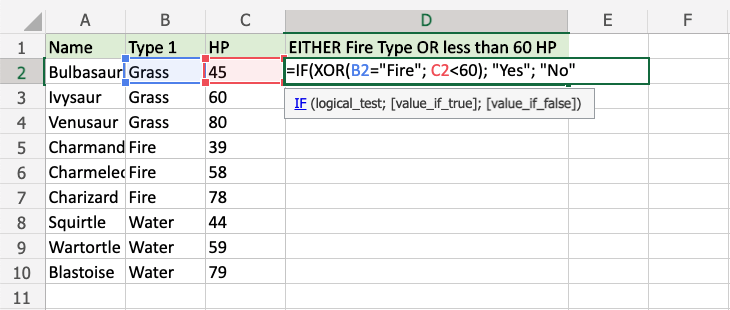The function can be repeated with the filling function for each row to perform the same check for each Pokemon: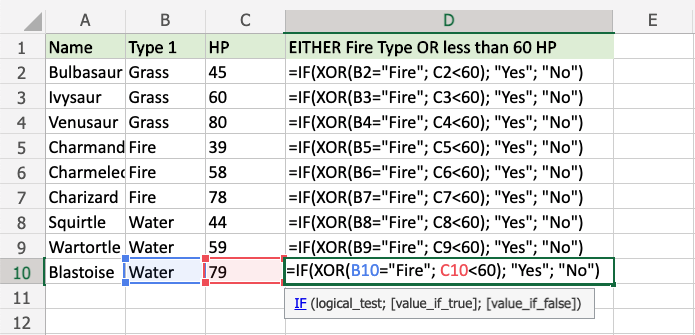Now, each row has a check for Water Type or Defense greater than 60, but not both: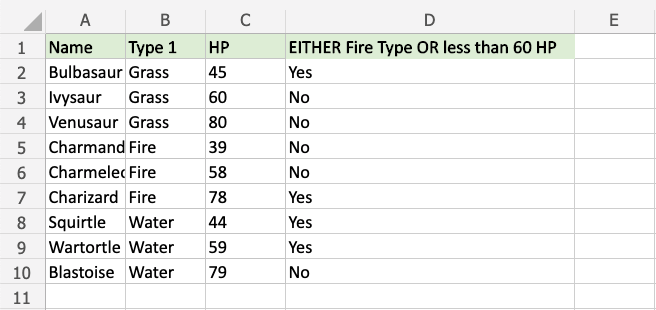For example:

• Ivysaur is not Fire type, but it also does not have less than 60 defense, so the function returns "No".
• Charmeleon is Fire type, and has less than 60 defense, so the function returns "No"
• Wartortle is not Fire type, but has less than 60 defense, so the function returns "Yes"